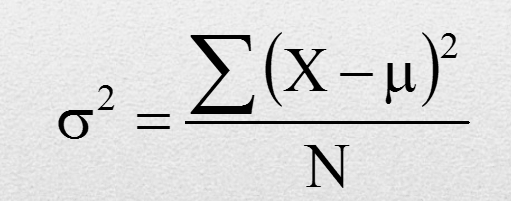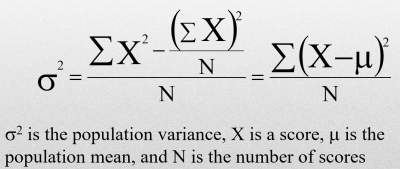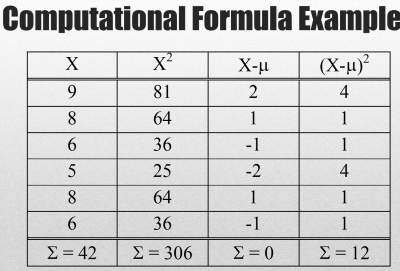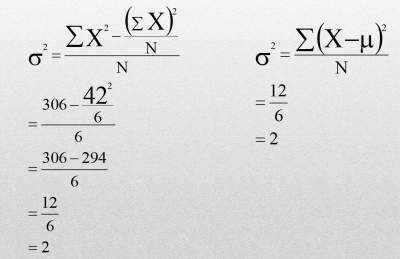# Measures of Central Tendencies for Ungrouped Data

## Mean, Mode, Median, and Range

Sure, the words "Mean, Median, Mode and Range" all sound confusing··· But what about the words we already know, like "Average, Middle, Most Frequent, and Difference"? They are all the same ideas!

Definition: What is the Mean?
The SUM of the data, divided by the number of items in the set of data··· commonly called "the average".

Definition: What is the Median?
The middle number in the set of data when written "in order"

Definition: What is the mode?
The data item that appears the "MOST"

Definition: What is the range?
It is a measure of dispersion that calculates the difference between the largest and smallest number in a set.

### Practical Exercise

The prices, in dollars, of the cars sold by a car salesperson in one week are shown below:
4300, 5400, 3200, 6200, 5400, 5500, 6400
Question:Find the mean, median, mode and range
1. Mean
2. Median
3. Mode
4. Range

Answers: Mean= 4314.3; Median = 5400; Mode = 5400; Range = 6400 - 3200 = 3200

### Variance

Definition: Variance is defined as the average of the square deviations as shown below:#### What Does the Variance Formula Mean?

• Variance is the mean of the squared deviation scores.
• The larger the variance is, the more the scores deviate.
• On average, away from the mean. The smaller the variance is, the less the scores deviate, on average, from the mean.

### Standard Deviation

The standard deviation is the square root of variance.
Variance = standard deviation2.

### Computational Formula

When calculating variance, it is often easier to use a computational formula which is algebraically equivalent to the definitional formula:An example is illstrated below: